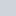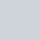### LQHosting – Two OpenVZ and Two KVM Plans, as low as \$50 a year!, , , , , , , , , , , , ,September 5, 2019 @ 9:00 am, by Frank WilliamsLQHosting, is back with some awesome OpenVZ and KVM offers for the community!
For those who desire minimal overhead, the OpenVZ VPS plan looks perfect. Those looking to run Docker or desire more control can consider the KVM VPS offer.
The following offers do not require any promotion code, as we’ve been told that they are custom order links created exclusively for LowEndBox.

They accept PayPal, Credit Cards, Alipay, Bitcoin, Litecoin, and Ethereum. You can find their ToS/Legal Docs here.

As always we hope you enjoy their promotions and if you have any comments/feedback, please be sure to share them in the comments section down below.

Here’s what they had to say:

“We aim to service every segment of the market ranging from individuals plus small to large businesses. To achieve this, we have set out on this mission and aim to achieve it with a mixture of quality service, 24×7 support, and flexible plans! Our servers are all connected to dual uplinks, with a redundant network blend. Additionally, all of the services that we offer are powered by SSD’s only as we are a performance-centric company. With a commitment to uptime and performance, LQHosting is the one and only source that can provide you with ultimate peace of mind!”

Here are the offers:

 1GB SSD KVM VPSCPU: 1 CoreRAM: 1024MBSSD Storage: 10GBBandwidth: 1500 GBVirtualization: KVM (Docker Supported!)Control Panel: SolusVMSetup: Instant\$50/yr[ORDER] 3GB SSD KVM VPSCPU: 3 CoresRAM: 3072MBSSD Storage: 40GBBandwidth: 10,000 GBVirtualization: KVM (Docker Supported!)Control Panel: SolusVMSetup: Instant\$60/yr[ORDER]
 2GB SSD OPENVZ VPSCPU: 2 CoresRAM: 2048MBSSD Storage: 30GBBandwidth: 3000 GBVirtualization: OpenVZControl Panel: SolusVMSetup: Instant\$90/yr[ORDER] 4GB SSD OPENVZ VPSCPU: 2 CoresRAM: 4096MBSSD Storage: 60GBBandwidth: 5000 GBVirtualization: OpenVZControl Panel: SolusVMSetup: Instant\$120/yr[ORDER]

NETWORK INFO:

Dallas, TX, USA

Test IPv4: 192.3.237.150

Test file: http://192.3.237.150/100MB.test

Los Angeles, CA, USA

Test IPv4: 107.175.180.6

Test file: http://107.175.180.6/100MB.test

OpenVZ VPS Nodes:

– Intel Xeon E3-1230v2

– 32GB RAM

– 4x 1TB Intel Enterprise SSD’s

– Hardware RAID-10

KVM VPS Nodes:

– Dual Intel Xeon E5-2650v2

– 192GB RAM

– 8x 1TB Intel Enterprise SSD’s

– Hardware RAID-10

### 1 Comment

1.Worked ok, when I bought last March. Since then s-l-o-w
Oversold ?? Supposedly SSD.

```----------------------------------------------------------------------
CPU model            : Intel(R) Xeon(R) CPU E3-1240 v3 @ 3.40GHz
Number of cores      : 2
CPU frequency        : 3392.006 MHz
Total size of Disk   : 40.0 GB (16.0 GB Used)
Total amount of Mem  : 3072 MB (32 MB Used)
Total amount of Swap : 3072 MB (0 MB Used)
System uptime        : 0 days, 16 hour 15 min
Load average         : 0.00, 0.01, 0.00
OS                   : Ubuntu 16.04.6 LTS
Arch                 : x86_64 (64 Bit)
Kernel               : 2.6.32-042stab138.1
----------------------------------------------------------------------
I/O speed(1st run)   : 80.6 MB/s
I/O speed(2nd run)   : 65.7 MB/s
I/O speed(3rd run)   : 60.2 MB/s
Average I/O speed    : 68.8 MB/s
----------------------------------------------------------------------
CacheFly                        205.234.175.175         6.96MB/s
Linode, Tokyo2, JP              139.162.65.37           3.38MB/s
Linode, Singapore, SG           139.162.23.4            3.70MB/s
Linode, London, UK              176.58.107.39           3.57MB/s
```
``` ssh lq fio seq_read.fio
myjob: (g=0): rw=read, bs=128K-128K/128K-128K/128K-128K, ioengine=libaio, iodepth=32
fio-2.2.10
Starting 1 process

myjob: (groupid=0, jobs=1): err= 0: pid=2786: Thu Sep 12 10:23:05 2019
read : io=1024.0MB, bw=123960KB/s, iops=968, runt=  8459msec
slat (usec): min=63, max=104223, avg=1019.62, stdev=5124.01
clat (usec): min=18, max=166663, avg=31851.30, stdev=24344.88
lat (usec): min=97, max=166765, avg=32871.73, stdev=24723.72
clat percentiles (msec):
|  1.00th=[    8],  5.00th=[    9], 10.00th=[   10], 20.00th=[   13],
| 30.00th=[   16], 40.00th=[   19], 50.00th=[   25], 60.00th=[   31],
| 70.00th=[   39], 80.00th=[   50], 90.00th=[   64], 95.00th=[   79],
| 99.00th=[  116], 99.50th=[  133], 99.90th=[  167], 99.95th=[  167],
| 99.99th=[  167]
bw (KB  /s): min=52321, max=269258, per=100.00%, avg=124999.81, stdev=67029.37
lat (usec) : 20=0.01%, 250=0.01%, 1000=0.02%
lat (msec) : 2=0.02%, 10=13.89%, 20=28.72%, 50=37.32%, 100=17.68%
lat (msec) : 250=2.32%
cpu          : usr=0.95%, sys=19.34%, ctx=564, majf=0, minf=545
IO depths    : 1=0.1%, 2=0.1%, 4=0.1%, 8=0.1%, 16=0.2%, 32=99.6%, >=64=0.0%
submit    : 0=0.0%, 4=100.0%, 8=0.0%, 16=0.0%, 32=0.0%, 64=0.0%, >=64=0.0%
complete  : 0=0.0%, 4=100.0%, 8=0.0%, 16=0.0%, 32=0.1%, 64=0.0%, >=64=0.0%
issued    : total=r=8192/w=0/d=0, short=r=0/w=0/d=0, drop=r=0/w=0/d=0
latency   : target=0, window=0, percentile=100.00%, depth=32

Run status group 0 (all jobs):
READ: io=1024.0MB, aggrb=123959KB/s, minb=123959KB/s, maxb=123959KB/s, mint=8459msec, maxt=8459msec
```

so 123MB in 8.5sec
comparable SPARKVPS:

``` ssh spark fio seq_read.fio
myjob: (g=0): rw=read, bs=(R) 128KiB-128KiB, (W) 128KiB-128KiB, (T) 128KiB-128KiB, ioengine=libaio, iodepth=32
fio-3.1
Starting 1 process

myjob: (groupid=0, jobs=1): err= 0: pid=24015: Thu Sep 12 10:25:34 2019
slat (usec): min=14, max=992, avg=40.27, stdev=49.28
clat (usec): min=542, max=75718, avg=2471.22, stdev=6327.24
lat (usec): min=585, max=75745, avg=2513.87, stdev=6332.42
clat percentiles (usec):
|  1.00th=[  955],  5.00th=[ 1123], 10.00th=[ 1205], 20.00th=[ 1336],
| 30.00th=[ 1418], 40.00th=[ 1516], 50.00th=[ 1647], 60.00th=[ 1795],
| 70.00th=[ 1926], 80.00th=[ 2073], 90.00th=[ 2278], 95.00th=[ 2671],
| 99.00th=, 99.50th=, 99.90th=, 99.95th=,
| 99.99th=
bw (  MiB/s): min= 1679, max= 1679, per=100.00%, avg=1679.00, stdev= 0.00, samples=1
iops        : min=13432, max=13432, avg=13432.00, stdev= 0.00, samples=1
lat (usec)   : 750=0.07%, 1000=1.43%
lat (msec)   : 2=73.82%, 4=21.09%, 10=1.75%, 20=0.95%, 50=0.15%
lat (msec)   : 100=0.74%
cpu          : usr=13.85%, sys=54.95%, ctx=126, majf=0, minf=1034
IO depths    : 1=0.1%, 2=0.1%, 4=0.1%, 8=0.1%, 16=0.2%, 32=99.6%, >=64=0.0%
submit    : 0=0.0%, 4=100.0%, 8=0.0%, 16=0.0%, 32=0.0%, 64=0.0%, >=64=0.0%
complete  : 0=0.0%, 4=100.0%, 8=0.0%, 16=0.0%, 32=0.1%, 64=0.0%, >=64=0.0%
issued rwt: total=8192,0,0, short=0,0,0, dropped=0,0,0
latency   : target=0, window=0, percentile=100.00%, depth=32

Run status group 0 (all jobs):
READ: bw=1556MiB/s (1632MB/s), 1556MiB/s-1556MiB/s (1632MB/s-1632MB/s), io=1024MiB (1074MB), run=658-658msec